Skewed or Imbalanced Dataset is the type of data in which a particular class or label is in high percentage as compared to other classes.

We should never train a machine learning model on skewed datasets because this might make our model overfit over the dominating class whereas neglecting all other minor classes which are low in amount.

Let us understand this by taking an example. We will analyze credit card data here.

Importing the libraries -

``````import numpy as np
import pandas as pd
import matplotlib.pyplot as plt
import seaborn as sns
``````

Importing the dataset and creating a data frame -

```data = pd.read_csv("creditcard.csv”)
```

Let us check what columns are there in the dataset.

```data.columns
```

Output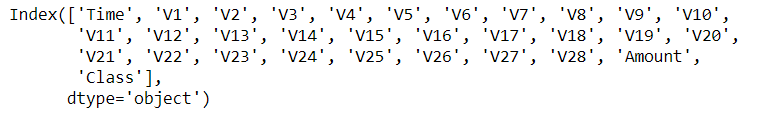```data["Class"].value_counts()
```

Output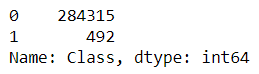Here the ‘Class’ feature is a categorical variable, with only values 0 and 1. “0” states “No fraud in Transaction” & “1” states “Fraud in Transaction”.

Let us calculate the percentage composition of each value in the “Class” feature.

```data["Class"].value_counts()/len(data)*100
```

Output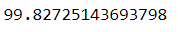```data["Class"].value_counts()/len(data)*100
```

Output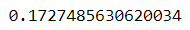We can see from the above calculations that the percentage of “No Fraud” is much higher than the percentage of “Fraud” in the dataset.

#### Visualization of the above data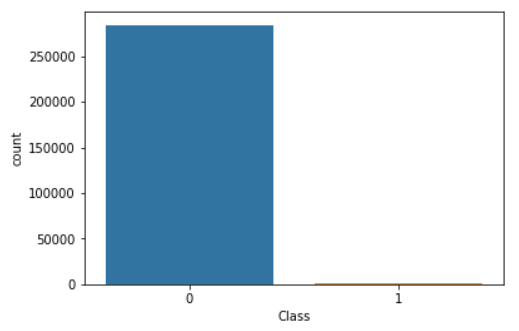When such a scenario appears where one of the class dominates much more than other classes, the dataset is said to be skewed or imbalanced. Such data should never be used for building the learning model because then the model will overfit for the dominating class and will not be able to prove to be a good fit for making predictions.

But what if, we have no other option than working with that imbalanced dataset. Is there something we can do to remove that skewness.

Yes, there is a method, by which we can create a more balanced dataset. We can use Random Undersampling for the “No fraud” class, which will basically remove data in order to create a more balanced dataset.

A dataset in which we have all the classes balanced in amount with each other will perform better in training the model without causing the model to overfit for any of the particular class in the dataset.